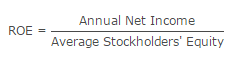# Return on Equity Ratio: Mathematical Explanation

Return on equity or return on capital is the ratio of net income of a business during a year to its stockholders’ equity during that year. It is a measure of profitability of stockholders’ investments. It shows net income as percentage of shareholder equity.

Formula

The formula to calculate return on equity is:Net income is the after tax income whereas average shareholders’ equity is calculated by dividing the sum of shareholders’ equity at the beginning and at the end of the year by 2. The net income figure is obtained from income statement and the shareholders’ equity is found on balance sheet. You will need year ending balance sheets of two consecutive financial years to find average shareholders’ equity.

Analysis

Return on equity is an important measure of the profitability of a company. Higher values are generally favorable meaning that the company is efficient in generating income on new investment. Investors should compare the ROE of different companies and also check the trend in ROE over time. However, relying solely on ROE for investment decisions is not safe. It can be artificially influenced by the management, for example, when debt financing is used to reduce share capital there will be an increase in ROE even if income remains constant.

Examples

Example 1: Company A earned net income of \$1,722,000 during the year ending march 31, 2011. The shareholders’ equity on April 30, 2010 and March 31, 2011 was \$14,587,000 and \$16,332,000 respectively. Calculate its return on equity for the year ending March 31, 2011.

Solution
Average Shareholders’ Equity = ( \$14,587,000 + \$16,332,000 ) / 2 = \$15,459,500
Return On Equity = \$1,722,000 / \$15,459,500 ≈ 0.11 or 11%

Example 2: Total assets and total liabilities of Company B on Jan 1, 2010 were \$2,342,000 and \$1,383,000. During the year ended December 31, 2011 it made a net profit of \$242,000 and its shareholders’ equity increased by \$302,000. Calculate ROE of Company B.

Solution
Step 1: Beginning Shareholders’ Equity = \$2,342,000 − \$1,383,000 = \$959,000
Step 2: Ending Shareholders’ Equity = \$959,000 + \$302,000 = \$1,261,000
Step 3: Average Shareholders’ Equity = ( \$959,000 + \$1,261,000 ) / 2 = \$1,110,000
Step 4: Return On Equity = \$242,000 / \$1,110,000 ≈ 0.22 or 22%